## Natural Equation

A natural equation is an equation which specifies a curve independent of any choice of coordinates or parameterization. The study of natural equations began with the following problem: given two functions of one parameter, find the Space Curve for which the functions are the Curvature and Torsion.

Eulergave an integral solution for plane curves (which always have Torsion). Call the Angle between the Tangent line to the curve and the x-Axis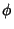the Tangential Angle, then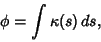(1)

whereis the Curvature. Then the equations(2)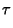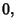(3)

whereis the Torsion, are solved by the curve with parametric equations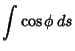(4)(5)

The equationsand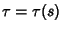are called the natural (or Intrinsic) equations of the space curve. An equation expressing a plane curve in terms ofand Radius of Curvature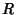(or) is called a Cesàro Equation, and an equation expressing a plane curve in terms ofandis called a Whewell Equation.

Among the special planar cases which can be solved in terms of elementary functions are the Circle, Logarithmic Spiral, Circle Involute, and Epicycloid. Enneper showed that each of these is the projection of a Helix on a Conic surface of revolution along the axis of symmetry. The above cases correspond to the Cylinder, Cone, Paraboloid, and Sphere.

References

Cesàro, E. Lezioni di Geometria Intrinseca. Napoli, Italy, 1896.

Euler, L. Comment. Acad. Petropolit. 8, 66-85, 1736.

Gray, A. Modern Differential Geometry of Curves and Surfaces. Boca Raton, FL: CRC Press, pp. 111-112, 1993.

Melzak, Z. A. Companion to Concrete Mathematics, Vol. 2. New York: Wiley, 1976.

Struik, D. J. Lectures on Classical Differential Geometry. New York: Dover, pp. 26-28, 1988.